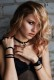# Grade 8 MTAP 2015 Elimination Questions with Solutions – Part 2

This is the second part (questions 11-20) of the solutions of the Grade 8 MTAP 2015 Elimination Questions. You can read the solutions for questions 1-10 here. If you found any errors in the solution, please comment in the box below.

11.) If$x = 4$ and$y = -3$, what is$x^2y + xy^2$?

Solution$x^2y + xy^2 = (4^2) (-3) + (4) (-3)^2 = (16) (-3) + 4(9)$$= (-48) + 36 = -12$

12.) Simplify$x(1 + y) - 2y(x - 2) + xy$

Solution$x(1 + y) - 2y (x - 2) + xy$$= x + xy - 2xy + 4y + xy$$= x + 2xy - 2xy + 4y$$= x + 4y$

Answer:$x + 4y$

13.) If$a$ and$b$ are positive constants, simplify$\dfrac{ab \sqrt{ab}}{\sqrt{a^4} \sqrt{b^3}}$.

Solution

Note that$\sqrt {ab} = (ab)^{1/2}$$\sqrt {a^4} = a^{4/3}$ and$\sqrt  {b^3} = b^{3/4}$

Now$\dfrac { (ab) (ab)^{1/2}} { a^{4/3} b^{3/4}}$$= \dfrac{ (ab) (a^{1/2}) (b^{1/2}) } {a^{4/3} b^{3/4}}$$=\dfrac {a^{3/2} b^{3/2}} {a^{4/3} b^{3/4}}$$= a^{(3/2 - 4/3)} b^{(3/2 - 3/4)}$$= a^{(9/6 - 8/6)} b^{(6/4 -3/4)}$$= a^{1/6}b^{3/4}$$a^{2/12}b^{9/12} = \sqrt  {a^2b^9}$

Answer:$a^{1/6}b^{3/4}$ or$\sqrt  {a^2b^9}$

14.) What is the quotient when$6x^4 + x^3 + 4x^2 + x + 2$ is divided by$3x^2 - x + 1$?

SolutionAnswer:$2x^2 + x + 1$ remainder$x + 1$.

15.) In Item 14, what is the remainder?

Answer:$x + 1$

16.) If$A + B = x - 2y$, what is$A^2 + 2AB + B^2 + 4xy$?

Solution$(A + B)^2 = (x-2y)^2$$A^2 + 2AB + B^2 = x^2 - 4xy + 4y^2$$A^2 + 2AB + B^2 + 4xy = x^2 - 4xy + 4xy + 4y^2$$A^2 + 2AB + B^2 + 4xy = x^2 + 4y^2$

Answer:$x^2 + 4y^2$

17.) If$x + y = 7$ and$xy = 5$, what is$x^3 + y^3$?

Solution$x + y = 7$ and$xy = 5$$(x + y)^3 = 7^3$$x^3 + 3x^2y + 3xy^2 + y^3 = 343$$x^3 + 3xy (x + y) + y^3 = 343$

Substituting the given values above,$x^3 + 3 (5)(7) + y^3 = 343$$x^3 + 105 + y^3 = 343$$x^3 + y^3 = 238$.

18.) If the length, width, and height of an open-top rectangular box are$(x + 3)$ cm,$x$ cm, and$(x - 3)$ cm, what is its surface area?

Solution

The formula for finding the surface area$S$ of a rectangular prism with length$l$, width$w$ and height$h$ is$S = 12lh + 2lw + 2wh$. Since the box is open, we subtract lw, which is the top face. So, the surface area of the open box is$S = 2lh + lw + 2wh$.

Substituting, we have$S = 2(x + 3) (x - 3) + x(x + 3) + 2x(x - 3)$$= 2(x^2 - 9) + x^2 + 3x + 2x^2 - 6x$$= 2x^2 - 18 + x^2 + 3x + 2x^2 - 6x$$=15x^2 - 3x - 18$

Answer:$15x^2 - 3x - 18$

19.) A man walked$x$ km for 2.5 hrs, then jogged$(2x + 3)$ km for 3.5 hrs, and finally walked again$(5x - 3)$ km for 4 hrs. If his average speed for the entire exercise was 4 kph, what is x?

Solution$\dfrac{2.5x + 3.5(2x + 3) + 4(5x -3)}{x + 2x + 5x} = 4$$\dfrac{29.5x - 1.5}{8x} = 4$$29.5x - 1.5 = 32x$$2.5x = 1.5$$x = 3/5 = 0.6$

20.) Simplify$(a - 3) (a + 3) (a^2 + 3a + 9) (a^2 - 3a + 9)$.

Solution

We can group the expressions as sum and difference of two cubes.$[(a - 3) (a^2 + 3a + 9)] [(a + 3) (a^2 - 3a + 9)]$$= (a^3 - 3^3) (a^3 + 3^3)$$= (a^3 - 27) (a^3 + 27)$

Now, this is in the form of the difference of two squares$(x + y) (x - y) = x^2 - y^2$.

So,$(a^3 - 27) (a^3 + 27) = (a^3)^2 - 27^2 = a^6 - 729$

Answer:$a^6 - 729$

### 7 responses to “Grade 8 MTAP 2015 Elimination Questions with Solutions – Part 2”

1.Jeaven Lozano

YOUR BERY HELP! EAT realy helps me to revyu my nowtes! Bery usepull

2.nogie

nice

3.Phoebe

18.5x^2-3x-18 dapat po

4.Phoebe

18.5x^2-3x-18 dapat po

5.ronnie aljibe

this will be of great help

6.bret romeo

Question about number 19, please help, i dont get it, the question is the value of x, since the only constant is the average speed for all the exercises, which is 4kph, can we just simply find the sum of all the distance traveled, divided by 10 and then equate it to 4?

7.desiree alyanna